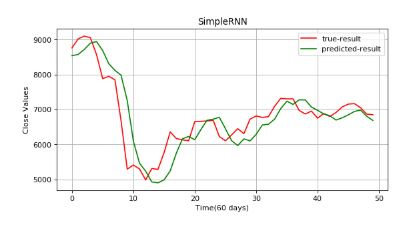Gaurav Singhal

# Getting Started with RNN

• Sep 9, 2020
• 3,328 Views
• Sep 9, 2020
• 3,328 Views
Data
Data Analytics
Machine Learning

## Introduction

Recurrent Neural Networks (RNNs) are a popular class of Artificial Neural Networks. RNNs are called recurrent because the output of each element of the sequence is dependent upon the previous computations.

When you watch a movie, you connect the dots because you know what happened in the last few scenes. Traditional NN architectures cannot do this. They cannot use previous scenes to predict what will happen in the next scene. Hence, to solve sequence-related problems, RNNs are used.

This tutorial will cover a brief introduction to simple RNNs and the concept of multi-layer perceptron (MLP). You will build a model on a time-series dataset to predict B value. Download the data here.

## Understanding MLP

MLP is a feed-forward neural network. It consists of three nodes: an input layer, a hidden layer, and an output layer. They are fully connected as each node in one layer connects to a certain weight to every node in the following layer. MLP uses forward propagation followed by a supervised learning technique called backpropagation for training. A representation of MLP is shown below.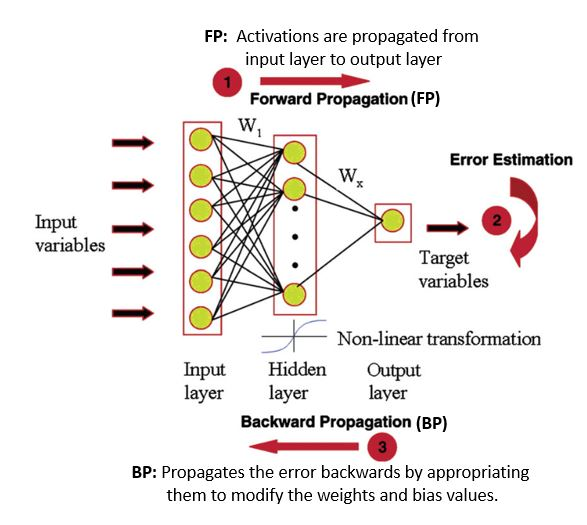If you are interested in knowing more about backpropagation, refer to this blog post.

## Understanding Simple RNNs

The idea behind an RNN is to make use of sequential information. The left side of the image below is a graphical illustration of the recurrence relation. The right part illustrates how the network unfolds through time over a sequence of length k. A typical unfolded RNN looks like this: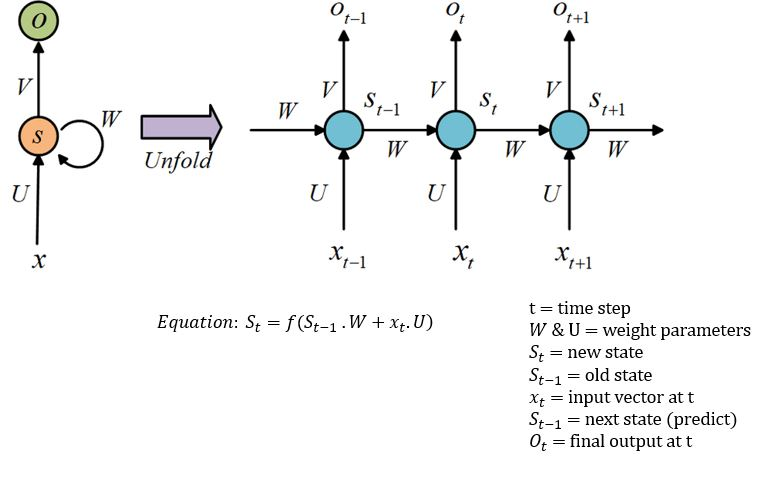The optimal parameters for this task are `U=W=1`. Let's train the RNN model.

## Understanding Backpropagation Through Time (BPTT) and Vanishing Gradient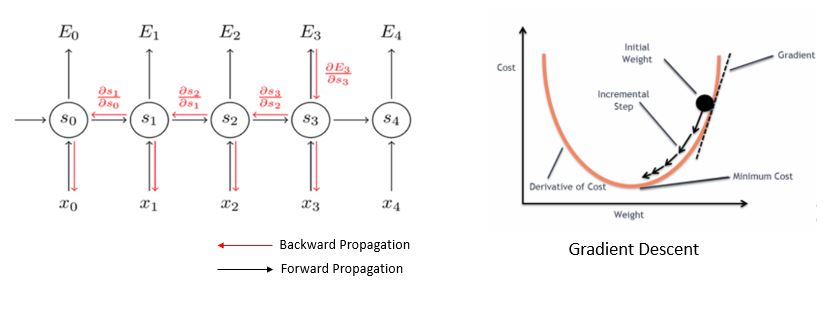To train the RNNs, BPTT is used. "Through time" is appended to the term "backpropagation" to specify that the algorithm is being applied to a temporal neural model (RNN). The task of BPTT is to find a local minimum, a point with the least error. By adjusting the values of weights, the network can reach minima. This process is called gradient descent. Gradients (steps) are computed by derivatives, partial derivatives, and chain rule.

But BPTT has difficulty learning long-term dependencies. You might suggest adding more RNNs. Theoretically, that is correct, but practically, it's the opposite. Stacks of RNNs give rise to the vanishing gradient problem. BPTT would make the gradient so small, effectively preventing weights from changing its value, that it would completely stop the NN from training further.

Let's implement the code.

## Code Implementation

You will be working with Bicton data, using 60 data points to predict the 61st data point.

``````1import numpy as np
2import pandas as pd
3import matplotlib.pyplot as plt
4import warnings
5from sklearn.metrics import mean_absolute_error
6from keras.models import Sequential
7from keras.layers import Dense, LSTM, Dropout,Flatten
8warnings.filterwarnings("ignore")``````
python

Add a column for `date` and convert `Timestamp` columns to date form.

``````1bit_data=pd.read_csv("../input/bitstampUSD.csv")
2bit_data["date"]=pd.to_datetime(bit_data["Timestamp"],unit="s").dt.date
3group=bit_data.groupby("date")
4data=group["Close"].mean()``````
python
``1data.shape``
python

The goal is to make a prediction of daily `close` data. The last 60 rows are considered the test dataset.

``````1close_train=data.iloc[:len(data)-60]
2close_test=data.iloc[len(close_train):]``````
python

Here values are set between 0-1 in order to avoid domination of high values.

``````1close_train=np.array(close_train)
2close_train=close_train.reshape(close_train.shape,1)
3from sklearn.preprocessing import MinMaxScaler
4scaler=MinMaxScaler(feature_range=(0,1))
5close_scaled=scaler.fit_transform(close_train)``````
python

Choose 60 data points as x-train and the 61st as y-train.

``````1timestep=60
2x_train=[]
3y_train=[]
4
5for i in range(timestep,close_scaled.shape):
6    x_train.append(close_scaled[i-timestep:i,0])
7    y_train.append(close_scaled[i,0])
8
9x_train,y_train=np.array(x_train),np.array(y_train)
10x_train=x_train.reshape(x_train.shape,x_train.shape,1) #reshaped for RNN
11print("x-train-shape= ",x_train.shape)
12print("y-train-shape= ",y_train.shape)``````
python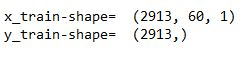## Implementation of MLP

The Keras `sequential()` API is used for creating the model. MLP is built on a stack of densely connected layers. Hence, the `dense()` function is added to extract important parameters. The first layer has 16 output neurons, and the next layer has eight outputs. Both are activated using ReLU.

Next, compile the architecture by adjusting the hyperparameters. Here, optimizer is used for optimizing our model and loss function. Then, fit the training data to the model with 50 epochs, or iterations.

``````1model = Sequential()
6
8model.fit(x_train,y_train,epochs=50,batch_size=64)``````
python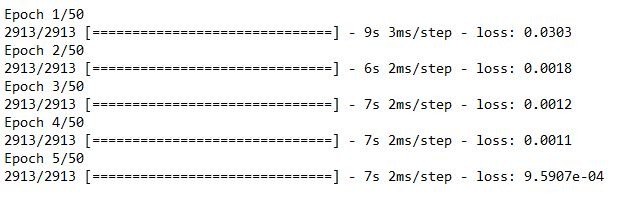Now the test data is been prepared for prediction.

``````1inputs=data[len(data)-len(close_test)-timestep:]
2inputs=inputs.values.reshape(-1,1)
3inputs=scaler.transform(inputs)``````
python
``````1x_test=[]
2for i in range(timestep,inputs.shape):
3    x_test.append(inputs[i-timestep:i,0])
4x_test=np.array(x_test)
5x_test=x_test.reshape(x_test.shape,x_test.shape,1)``````
python

Let's apply the model on the test data.

``````1predicted_data=model.predict(x_test)
2predicted_data=scaler.inverse_transform(predicted_data)``````
python
``````1data_test=np.array(close_test)
2data_test=data_test.reshape(len(data_test),1)``````
python

Plot the predictions.

``````1plt.figure(figsize=(8,4), dpi=80, facecolor='w', edgecolor='k')
2plt.plot(data_test,color="r",label="true result")
3plt.plot(predicted_data,color="b",label="predicted result")
4plt.legend()
5plt.xlabel("Time(60 days)")
6plt.ylabel("Values")
7plt.grid(True)
8plt.show()``````
python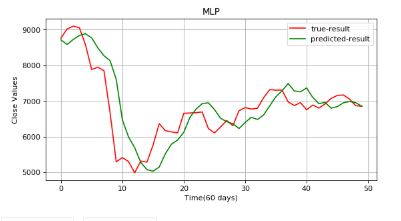There is a huge gap between the true value and predicted results. The results aren't reliable. Let's implement RNN.

## Implementation of Simple RNN

SimpleRNN will have a 2D tensor of shape (batch_size, internal_units) and an activation function of `relu`. As discussed earlier, RNN passes information through the hidden state, so let's keep true. A dropout layer is added after every layer. The matrix will be converted into one column using `Flatten()`. Lastly, compile the model.

``````1reg=Sequential()
10
12reg.fit(x_train,y_train,epochs=50,batch_size=64)``````
python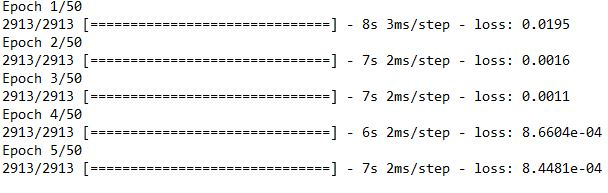it's time to predict.

``````1predicted_data=reg.predict(x_test)
2predicted_data=scaler.inverse_transform(predicted_data)``````
python
``````1plt.figure(figsize=(8,4), dpi=80, facecolor='w', edgecolor='k')
2plt.plot(data_test,color="r",label="true-result")
3plt.plot(predicted_data,color="g",label="predicted-result")
4plt.legend()
5plt.xlabel("Time(60 days)")
6plt.ylabel("Close Values")
7plt.grid(True)
8plt.show()``````
python